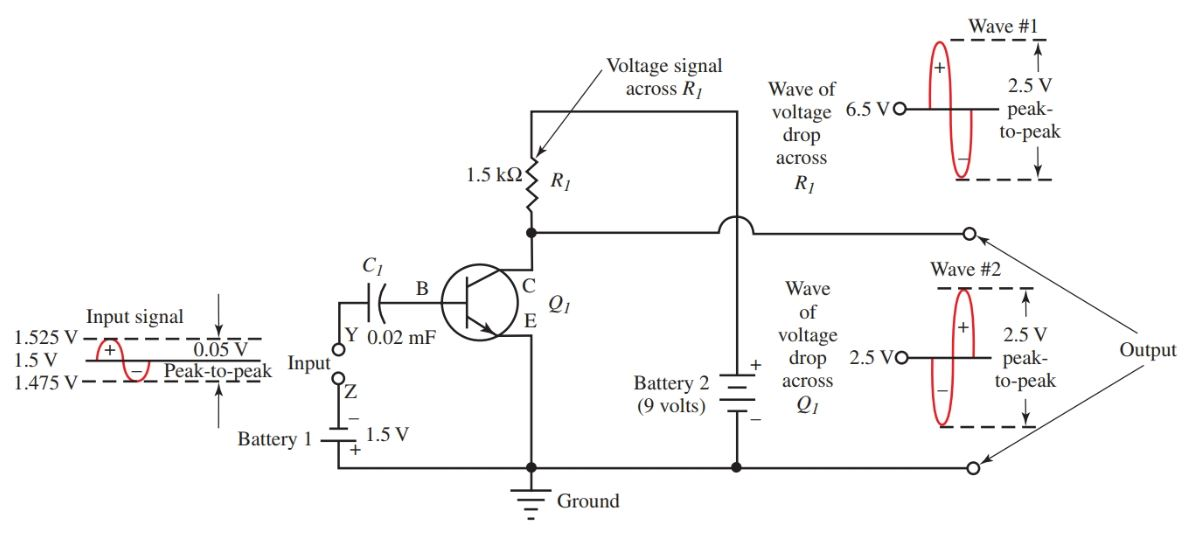# Common Emitter Amplifier: Working & Circuit Diagram

Want create site? Find Free WordPress Themes and plugins.

Amplifier Definition: An amplifier is an electronic device that uses a small input signal (voltage or current) to control a larger output signal. This principle works best when the output load, or impedance, of the circuit, is greater than the input load.

Examine Figure 1. The input is a microphone in the emitter and base control circuit. The output is a loudspeaker connected to the collector and emitter circuit.

The load of the loudspeaker is much greater than that of the microphone. The output current in the circuit is about the same as the input current.

Recalling Ohm’s law, if the input and output currents are about the same, and the resistance (load or impedance) is 50 times greater in the output than in the input, then the output voltage will be 50 times as great as the input signal. This process is signal amplification.Figure 1. Input circuit controlling output circuit (signal) in an NPN Common-Emitter amplifier.

Refer to Figure 2. It shows an NPN Common-Emitter amplifier circuit with actual component values and specific voltages. The emitter is common to both the input and output circuits.

The ac input signal is impressed across the emitter and base of the transistor Q1. The output signal is taken across the collector and emitter of Q1.

A capacitor, C1, is placed in the input signal to the couple (or pass) the ac input signal to the base for control. A ground is added at a common connection in the battery 1, emitter, and the battery 2 circuit.Figure 2. Common emitter NPN amplifier circuit diagram

• A 0.05 volt, peak-to-peak, ac input signal is applied across the emitter to base at points Y and Z. As the signal swings positive, the base-to-emitter forward bias voltage (signal plus bias) will increase.
• When the forward bias voltage increases, the base current will also increase resulting in a smaller resistance across the emitter-to-collector of transistor Q1.
• The smaller resistance causes the current in the circuit made of battery 2, R1, and the emitter/collector path of Q1, to increase. This increase in current through a fixed value resistor, R1, causes a greater voltage drop across the resistor. (See wave #1.)
• The increase in voltage drop across R1 causes a decrease in voltage across Q1 because they are both in series with the 9-volt battery, battery 2. (See wave #2.)
• Keep in mind the resulting voltage in a series circuit. The total voltage, EB2, is equal to the sum of the voltage drops in that series circuit, including the transistor, Q1, and the resistor, R1.
• During the negative half of the input signal, the bias voltage on the base is decreased or lowered. As a result, the resistance of the emitter-to-collector will increase causing current to be reduced.
• If the current flowing in the emitter-to-collector circuit is decreased, current will also decrease in R1, since it is in series with the collector of Q1.
•  If the current through a fixed value resistor, R1, is decreased, the voltage drop across it will also decrease. (See the negative half of wave #1.) This decrease in voltage across R1 causes an increase in voltage drop across the emitter-to-collector of Q1 as the sine wave swings positive in wave #2.

In any case, both wave #1 and wave #2 must add up to nine volts, Figure 3. Note that wave # 1 and wave #2 are 180 degrees out of phase.

A 0.05 volt signal in the input circuit (emitter-to- base in Q1) has controlled a 2.5 volt signal in the output (emitter-to-collector in Q1). This is amplification.Figure 3. Relationship of an output signal and ac signal across R1.

Computing Gain

There are a number of formulas that can be used to compute types of amplification in transistor amplifier circuits. When the formulas show a triangle, or delta (Δ), symbol, it means “the change in” a certain value or waveform.

Voltage Gain

The voltage gain (AV) is calculated by dividing the change in the output voltage by the change in the input voltage.

${{A}_{V}}=\frac{\Delta {{E}_{out}}}{\Delta {{E}_{in}}}=\frac{\Delta {{E}_{out\text{ }p-p}}}{\Delta {{E}_{in\text{ }p-p}}}$

Current Gain

The current gain (AI) is calculated by dividing the change in the output current by the change in the input current.

${{A}_{I}}=\frac{\Delta {{I}_{out}}}{\Delta {{I}_{in}}}$

Power gain

The power gain (AP) is calculated like Watt’s law. The voltage gain (AV) is multiplied by the current gain (AI).

${{A}_{P}}={{A}_{V}}\times {{A}_{I}}$

Gain is a ratio and, therefore, a dimensionless number. Gain simply tells how much a signal has been amplified. The value is a number, like 100, meaning the voltage gain is 100 times larger in the output than in the input.

Decibel Definition

A decibel (dB) is a unit of relative measurement. When a measurement is definitive, it has a definite weight, size, volume, etc. When a measurement is relative, it must be compared to a similar, known object.

Compare two different shades of blue, such as the blue sky and the blue water. There is no definite measurement as to just how the blue sky is or how blue the water is. However, we can compare the shades of the sky and water to determine which is bluer.

The decibel was developed as a way to measure the relativity of sound or to compare the level of one sound to another.

In the field of electronics, audio systems are expressed in decibels. Many times amplifiers are also classified in terms of decibels.

Instead of having a definitive output, the output of the sound system or amplifier is expressed in decibels. This is a way of saying that the output is 10, 100, or 1000 or more times greater than the input. A decibel rating gives relative power level and not an actual power rating.

Did you find apk for android? You can find new Free Android Games and apps.